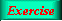Lecture 2 - Page 22 : 46
 Functional Programming in SchemeExpressions, Types, and Functions * Lisp and Scheme Lisp Scheme * Expressions and values Expressions, values, and types Examples of expressions and their values Evaluation of parenthesized expressions Arithmetic expressions Equality in Scheme The read-eval-print loop * Types Types Type checking Static type checking An example of type checking Types in functional programming languages * Lists Proper lists Symbolic expressions and improper lists Practical list construction List functions Association lists Property lists Tables as lists of rows Programs represented as lists * Other Data Types Other simple types Vectors Strings * Definitions Definitions * Functions The function concept Lambda calculus Functions in Scheme Function objects Functions as first class values Anonymous functions Lambda expressions in Scheme Optional parameters of Scheme functions (1) Optional parameters of Scheme functions (2) Closures Function definition in Scheme Simple web-related functions (1) Simple web-related functions (2) Function exercises
 Association lists
 An association list is a list of cons pairs Association lists are used in the same way as associative arrays
 Expression Value ```(define computer-prefs '((peter . windows) (lars . mac) (paw . linux) (kurt . unix)))``` `(assq 'lars computer-prefs)` `(lars . mac)` `(assq 'kurt computer-prefs)` `(kurt . unix)` ```(define computer-prefs-1 (cons (cons 'lene 'windows) computer-prefs))``` `computer-prefs-1` ```((lene . windows) (peter . windows) (lars . mac) (paw . linux) (kurt . unix))```

Examples of association lists. The function assq uses eq? to compare the first parameter with the first element - the key element - in the pairs. As an alternative, we could use the function assoc, which uses equal? for comparison. A better and more general solution would be to pass the comparison function as parameter. Notice in this context, that both assq and assoc are 'traditional Lisp functions' and part of Scheme, as defined in the language report.Creation of association listsAssociation list and property lists
 Associative arrays - OOP (in Danish)